Courses

# Solutions (Chapter Test - Non-Medical)

## 20 Questions MCQ Test Chemistry for IIT JEE (Main & Advanced) | Solutions (Chapter Test - Non-Medical)

Description
This mock test of Solutions (Chapter Test - Non-Medical) for Class 12 helps you for every Class 12 entrance exam. This contains 20 Multiple Choice Questions for Class 12 Solutions (Chapter Test - Non-Medical) (mcq) to study with solutions a complete question bank. The solved questions answers in this Solutions (Chapter Test - Non-Medical) quiz give you a good mix of easy questions and tough questions. Class 12 students definitely take this Solutions (Chapter Test - Non-Medical) exercise for a better result in the exam. You can find other Solutions (Chapter Test - Non-Medical) extra questions, long questions & short questions for Class 12 on EduRev as well by searching above.
QUESTION: 1

Solution:
QUESTION: 2

Solution:
QUESTION: 3

### Elevation in boiling point was 0.52°C when 6 g of a compound X was dissolved in 100 g of water. Molecular weight of X is (Kb for H2O is 0.52)

Solution:
QUESTION: 4

0.5 molal aqueous solution of a weak acid(HX) is 20% ionised. If Kf for water is 1.86 K kg mol–1, the lowering in freezing point of the solution is

Solution:
QUESTION: 5

Two beakers have equal volumes of solutions. One contains 60 g of glucose and other contains 60 g of
urea. Which one of the following is true about two solutions ?

Solution:
QUESTION: 6

For an ideal solution with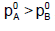, which of the following is true ?

Solution:
QUESTION: 7

Two beakers of capacity 500 mL were taken. One of these beakers, labelled as “A”, was filled with
400 mL water whereas the beaker labelled “B” was filled with 400 mL of 2 M solution of NaCl. At the same
temperature both the beakers were placed in closed containers of same material and same capacity as in
figure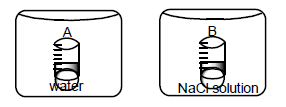At the given temperature, which of the following statement is correct about the vapour pressure of pure
water and that of NaCl solution.

Solution:
QUESTION: 8

The vapour pressure of a pure liquid ‘A’ is 70 torr at 27°C. It forms an ideal solution with another liquid B.
The mole fraction of B is 0.2 and total pressure of the solution is 84 torr at 27°C. The vapour pressure of pure
liquid B at 27°C is

Solution:
QUESTION: 9

A 0.004 M solution of Na2SO4 is isotonic with a 0.010 M solution of glucose at same temperature. The apparent degree of dissociation of Na2SO4 is

Solution:
QUESTION: 10

At 40°C, the vapour pressure in torr of methanol and ethanol solution is P = 119x + 135 (where x is the mole
fraction of methanol).Hence,

Solution:
QUESTION: 11

Concentrated aqueous sulphuric acid is 98% H2SO4 by mass and has a density of 1.80 g mol–1. The volume
of acid required to make one litre of 0.1 M H2SO4 solution

Solution:
QUESTION: 12

Two liquids A and B have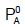and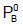in the ratio of 1 : 3. If the ratio of number of moles of A and B are
1 : 3, the mole fraction of ‘A’ in vapour phase in equilibrium with the solution is equal to

Solution:
QUESTION: 13

The molal elevation constant of water is 0.51. The boiling point of 0.1 molal aqueous NaCl solution is
nearly

Solution:
QUESTION: 14

The freezing point of a solution prepared from 1.25 g of non-electrolyte and 20 g of water is 271.9 K. If
molar depression constant is 1.86 K mol–1 then molar mass of the solute will be

Solution:
QUESTION: 15

An aqueous solution freezes at –0.186°C,what will be elevation in boiling point of the solution? (Kf = 1.860,
Kb = 0.512°)

Solution:
QUESTION: 16

Benzene and toluene form nearly ideal solutions. At 20o C, the vapour pressure of benzene is 75 torr and that of toluene is 22 torr. The partial vapour pressure of benzene at 20 C for a solution containing 78 g of benzene and 46 g of toluene in torr is

Solution:
QUESTION: 17

When a solute B is added to a solution of A then solution appears hotter. Which of the following graph correctly represent nature of solution ?

Solution:
QUESTION: 18

How many gram of dibasic acid(mol. mass 200) should be present in 100 mL of the aqueous solution to give
0.1 N solution ?

Solution:
QUESTION: 19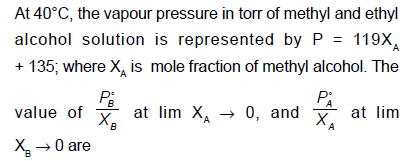Solution:
QUESTION: 20

At 800C, the vapour pressure of pure liquid ‘A’ is 520 mm Hg and that of pure liquid ‘B’ is 1000 mm Hg. If a
mixture solution of ‘A’ and ‘B’ boils at 800C and 1 atm pressure , then amount of ‘A’ in the mixture is (1 atm =
760 mm Hg)

Solution: